# MA.912.AR.5.4Export Print
Write an exponential function to represent a relationship between two quantities from a graph, a written description or a table of values within a mathematical or real-world context.

### Clarifications

Clarification 1: Within the Algebra 1 course, exponential functions are limited to the forms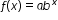, where b is a whole number greater than 1 or a unit fraction, or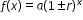, where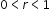.

Clarification 2: Within the Algebra 1 course, tables are limited to having successive nonnegative integer inputs so that the function may be determined by finding ratios between successive outputs.

General Information
Subject Area: Mathematics (B.E.S.T.)
Strand: Algebraic Reasoning
Status: State Board Approved

## Benchmark Instructional Guide

• Exponential

### Vertical Alignment

Previous Benchmarks

Next Benchmarks

### Purpose and Instructional Strategies

In middle grades, students solved problems involving percentages, including percent increases and decreases and write equations that represent proportional relationships. In Algebra I, students write exponential functions that model relationships characterized by having a constant percent of change per unit interval. In later courses, students will further develop their understanding of this feature of exponential functions.

• Provide opportunities to reference MA.912.AR.1.1 as students identify and interpret parts of an exponential equation or expression as growth or decay and connect them to key features of the graph.
• Problems include cases where the initial value is not given.
• Instruction includes guidance on how to determine the initial value or the percent rate of change of an exponential function when it is not provided.
• For example, if the initial value of (0,3) is given, students can now write the function as $f$($x$) = 3$b$$x$  . Guide students to choose a point on the curve that has integer coordinates such as (2, 12). Lead them to substitute the point into their function to find $b$. Students should recognize that exponential functions are restricted to positive values of $b$, leading to the function $f$($x$) = 3(2)$x$ .
• Instruction includes interpreting percentages of growth/decay from exponential functions expressed in the form $f$($x$) = $a$$b$$x$and see that $b$ can be used to determine a percentage.
• For example, the function $f$($x$) = 500(0.72) represents 16% growth of an initial value.
• Guide students to discuss the meaning of the number 1.16 as a percent. They should understand it represents 116%. Taking 116% of an initial value increases the magnitude of that value. (Students can test this in a calculator to confirm.) Taking this percentage repetitively leads to exponential growth.
• For example, the function $f$($x$) = 500(0.72)$x$  represents 28% decay of an initial value.
• Guide students to discuss the meaning of the number 0.72 as a percent. They should understand it represents 72%. Taking 72% of an initial value decreases the magnitude of that value. (Students can test this in a calculator to confirm.) Taking this percentage repetitively leads to exponential decay.
• For example, the function $f$($x$) = 500(1)$x$ represents an initial value that neither grows nor decays as $x$ increases.
• Guide students to discuss the meaning of the number 1 when it comes to growth/decay factors. They should understand it represents 100%. Taking 100% of an initial value causes the value to remain the same. (Students can test this in a calculator to confirm.) Taking this percentage repetitively leads to no change in the initial value (explaining the horizontal line that shows when $b$ = 1 on the graph).

### Common Misconceptions or Errors

• Students may not understand that exponential function values will eventually get larger than those of any other polynomial functions because they do not fully understand the impact of exponents on a value.

### Strategies to Support Tiered Instruction

• Teacher provides students with a graphic displaying key terms within an exponential function.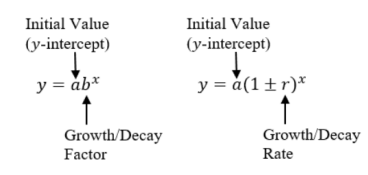• Instruction includes comparing quadratic (polynomial) to exponential functions using graphs or tables with whole-number inputs to show how exponential functions will quickly exceed quadratic (polynomial) functions.

Instructional Task 1 (MTR.4.1, MTR.5.1, MTR.7.1
• Karl and Simone were working on separate biology experiments. Each student documented their cell population counts over time in the chart below.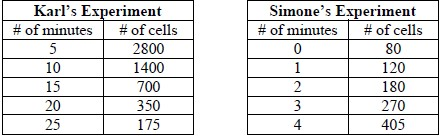• Part A. Do the number of cells in Simone’s experiment increase at a constant percentage rate of change? If so, what is the percentage rate? If not, describe what is happening to the number of cells. Does this change represent growth or decay? Justify your answer.
• Part B. Write exponential functions to represent the relationship between the quantities for each student’s experiment. In which experiment are the number of cells changing more rapidly? Justify your answer.
• Part C. Graph these functions and determine their key features.

• The population of J-Town in 2019 was estimated to be 76,500 people with an annual rate of increase of 2.4%.
• Part A. Write an equation to model future growth.
• Part B. What is the growth factor for J-Town?
• Part C. Use the equation to estimate the population in 2072 to the nearest hundred people.

### Instructional Items

Instructional Item 1
• Write an exponential function that represents the graph below.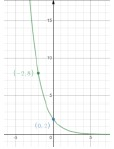Instructional Item 2

• A forester has determined that the number of fir trees in a forest is decreasing by 3% per year. In 2010, there were 13,000 fir trees in the forest. Write an equation that represents the number of fir trees, $N$, in terms of $t$, the number of years since 2010.

*The strategies, tasks and items included in the B1G-M are examples and should not be considered comprehensive.

## Related Courses

This benchmark is part of these courses.
1200310: Algebra 1 (Specifically in versions: 2014 - 2015, 2015 - 2022, 2022 and beyond (current))
1200320: Algebra 1 Honors (Specifically in versions: 2014 - 2015, 2015 - 2022, 2022 and beyond (current))
1200330: Algebra 2 (Specifically in versions: 2014 - 2015, 2015 - 2022, 2022 and beyond (current))
1200340: Algebra 2 Honors (Specifically in versions: 2014 - 2015, 2015 - 2022, 2022 and beyond (current))
1200380: Algebra 1-B (Specifically in versions: 2014 - 2015, 2015 - 2022, 2022 and beyond (current))
1200400: Foundational Skills in Mathematics 9-12 (Specifically in versions: 2014 - 2015, 2015 - 2022, 2022 and beyond (current))
7912070: Access Mathematics for Liberal Arts (Specifically in versions: 2014 - 2015, 2015 - 2018, 2018 - 2019, 2019 - 2022, 2022 - 2023, 2023 and beyond (current))
7912090: Access Algebra 1B (Specifically in versions: 2014 - 2015, 2015 - 2018, 2018 - 2019, 2019 - 2022, 2022 and beyond (current))
1200315: Algebra 1 for Credit Recovery (Specifically in versions: 2014 - 2015, 2015 - 2022, 2022 and beyond (current))
1200385: Algebra 1-B for Credit Recovery (Specifically in versions: 2014 - 2015, 2015 - 2022, 2022 and beyond (current))
7912075: Access Algebra 1 (Specifically in versions: 2014 - 2015, 2015 - 2018, 2018 - 2019, 2019 - 2022, 2022 and beyond (current))
7912095: Access Algebra 2 (Specifically in versions: 2016 - 2018, 2018 - 2019, 2019 - 2022, 2022 and beyond (current))
1207350: Mathematics for College Liberal Arts (Specifically in versions: 2022 and beyond (current))
1200710: Mathematics for College Algebra (Specifically in versions: 2022 and beyond (current))

## Related Access Points

Alternate version of this benchmark for students with significant cognitive disabilities.
MA.912.AR.5.AP.4: Select an exponential function to represent two quantities from a graph or a table of values.

## Related Resources

Vetted resources educators can use to teach the concepts and skills in this benchmark.

## Formative Assessments

Students are asked to write and solve an equation that models an exponential relationship between two variables.

Type: Formative Assessment

Case In Point:

Students are asked to explain the relationship between the set of solutions and the graph of an exponential equation.

Type: Formative Assessment

What Is the Function Rule?:

Students are asked to write function rules for sequences given tables of values.

Type: Formative Assessment

Writing an Exponential Function From a Table:

Students are asked to write an exponential function represented by a table of values.

Type: Formative Assessment

Writing an Exponential Function From a Description:

Students are asked to write an exponential function from a written description of an exponential relationship.

Type: Formative Assessment

Writing an Exponential Function From Its Graph:

Students are asked to write an exponential function given its graph.

Type: Formative Assessment

## Lesson Plan

Which Function?:

This activity has students apply their knowledge to distinguish between numerical data that can be modeled in linear or exponential forms. Students will create mathematical models (graph, equation) that represent the data and compare these models in terms of the information they show and their limitations. Students will use the models to compute additional information to predict future outcomes and make conjectures based on these predictions.

Type: Lesson Plan

## Original Student Tutorial

Creating Exponential Functions:

Follow as we construct an exponential function from a graph, from a table of values, and from a description of a relationship in the real world in this interactive tutorial.

Type: Original Student Tutorial

## MFAS Formative Assessments

Case In Point:

Students are asked to explain the relationship between the set of solutions and the graph of an exponential equation.

Students are asked to write and solve an equation that models an exponential relationship between two variables.

What Is the Function Rule?:

Students are asked to write function rules for sequences given tables of values.

Writing an Exponential Function From a Description:

Students are asked to write an exponential function from a written description of an exponential relationship.

Writing an Exponential Function From a Table:

Students are asked to write an exponential function represented by a table of values.

Writing an Exponential Function From Its Graph:

Students are asked to write an exponential function given its graph.

## Original Student Tutorials Mathematics - Grades 9-12

Creating Exponential Functions:

Follow as we construct an exponential function from a graph, from a table of values, and from a description of a relationship in the real world in this interactive tutorial.

## Student Resources

Vetted resources students can use to learn the concepts and skills in this benchmark.

## Original Student Tutorial

Creating Exponential Functions:

Follow as we construct an exponential function from a graph, from a table of values, and from a description of a relationship in the real world in this interactive tutorial.

Type: Original Student Tutorial

## Parent Resources

Vetted resources caregivers can use to help students learn the concepts and skills in this benchmark.